Examples

Chapter 8 Class 11 Sequences and Series
Serial order wise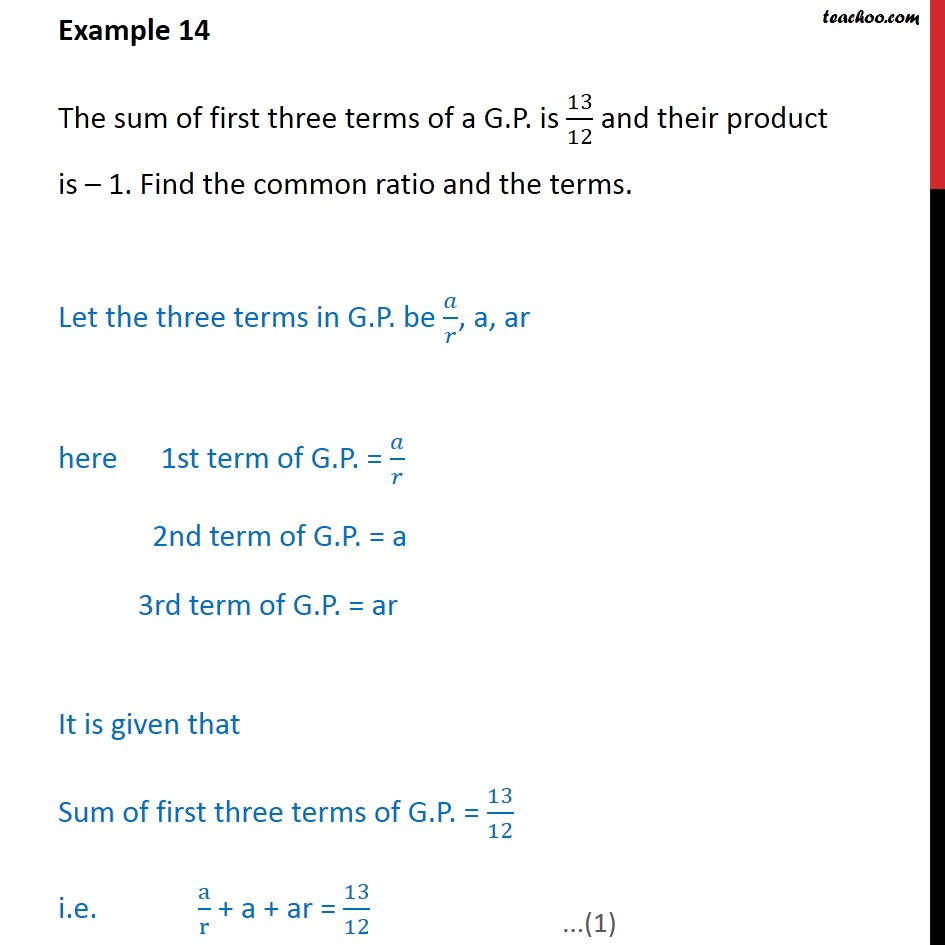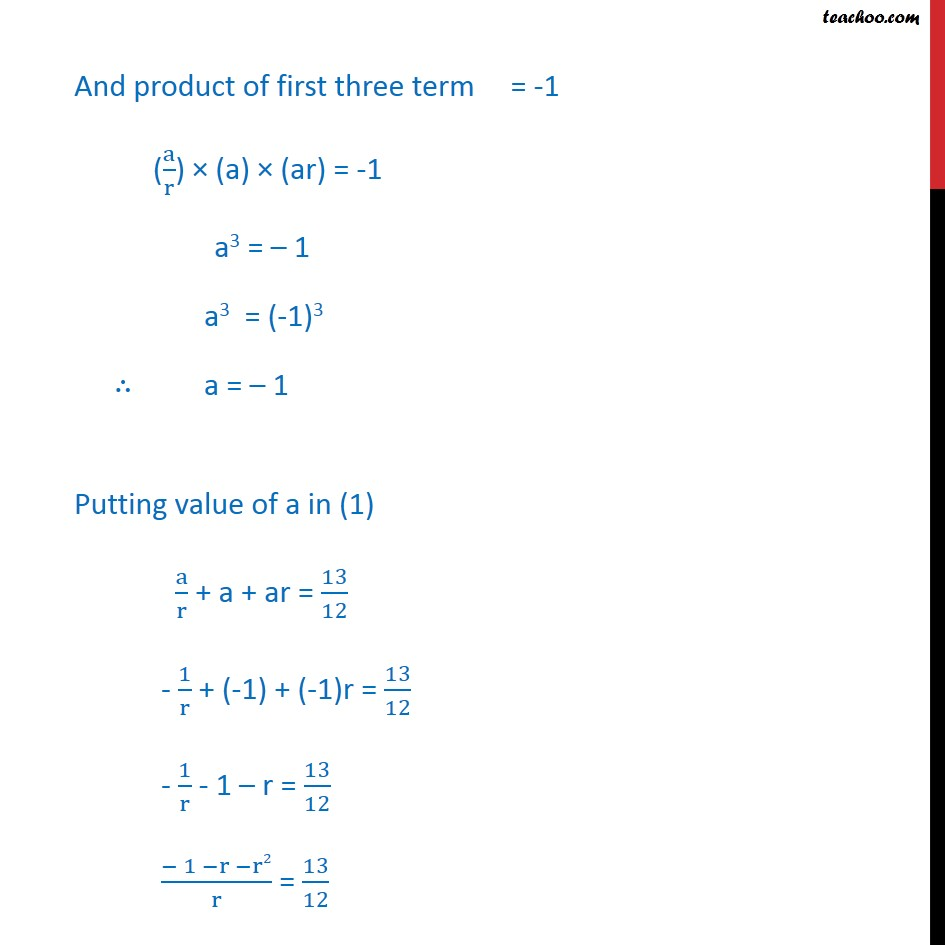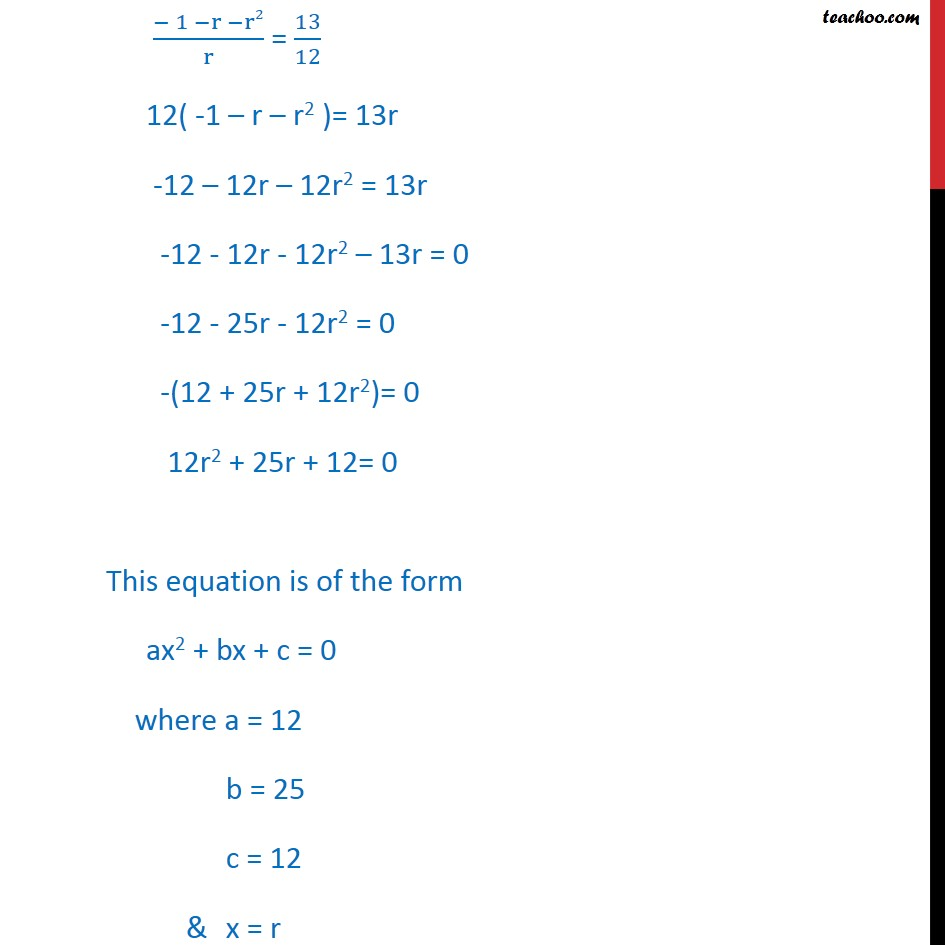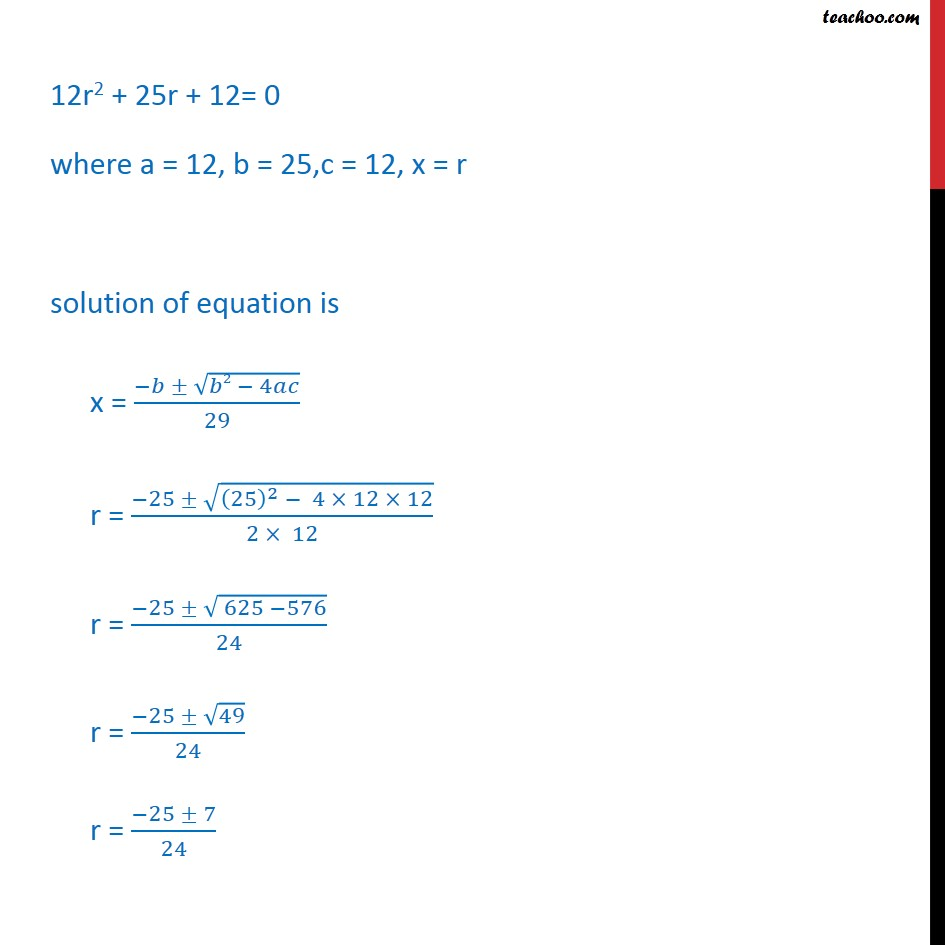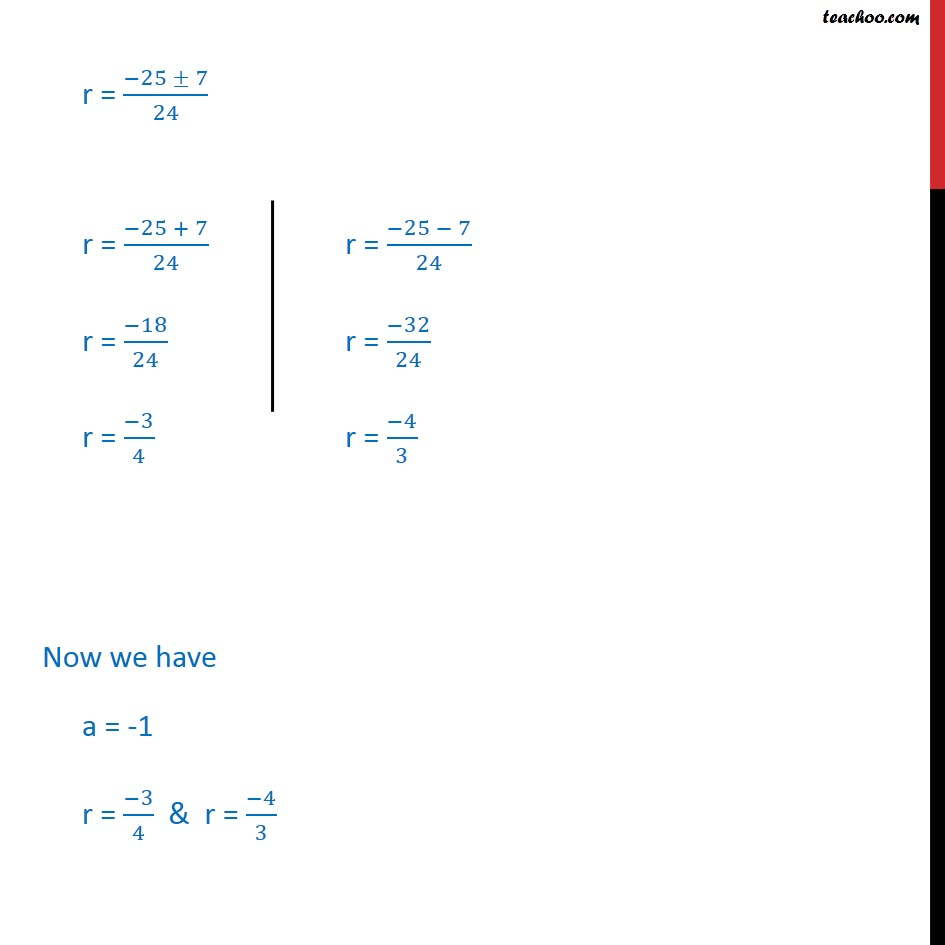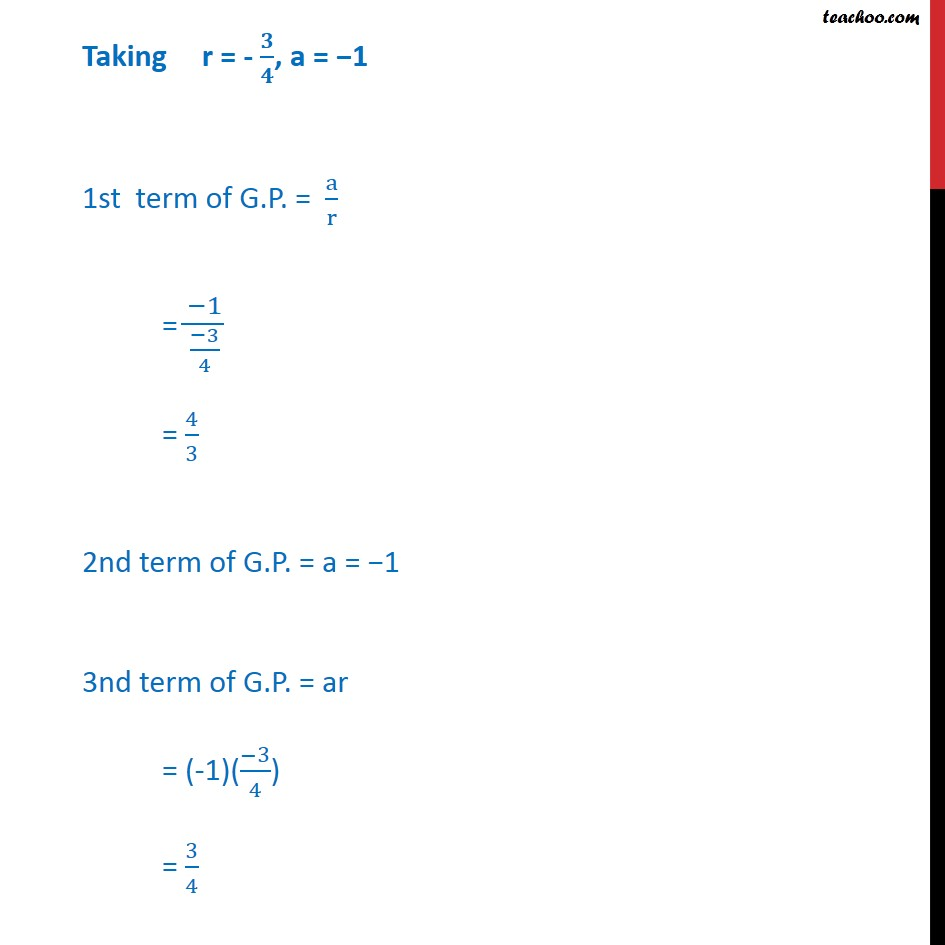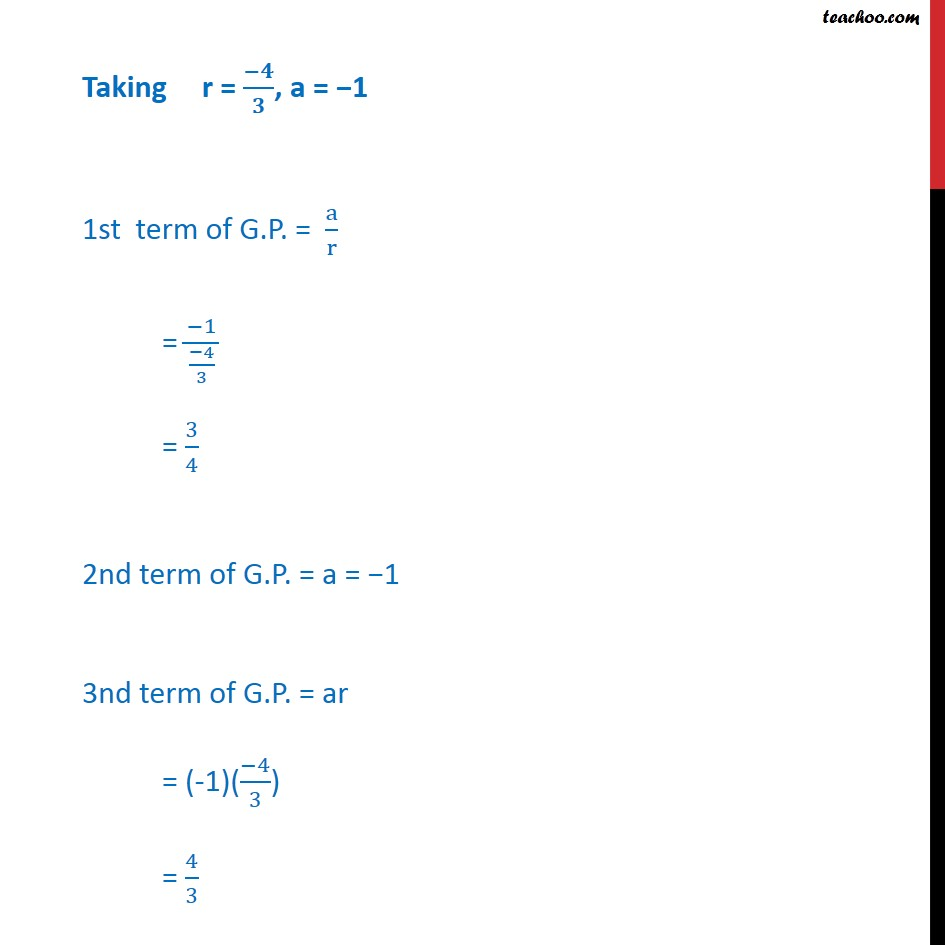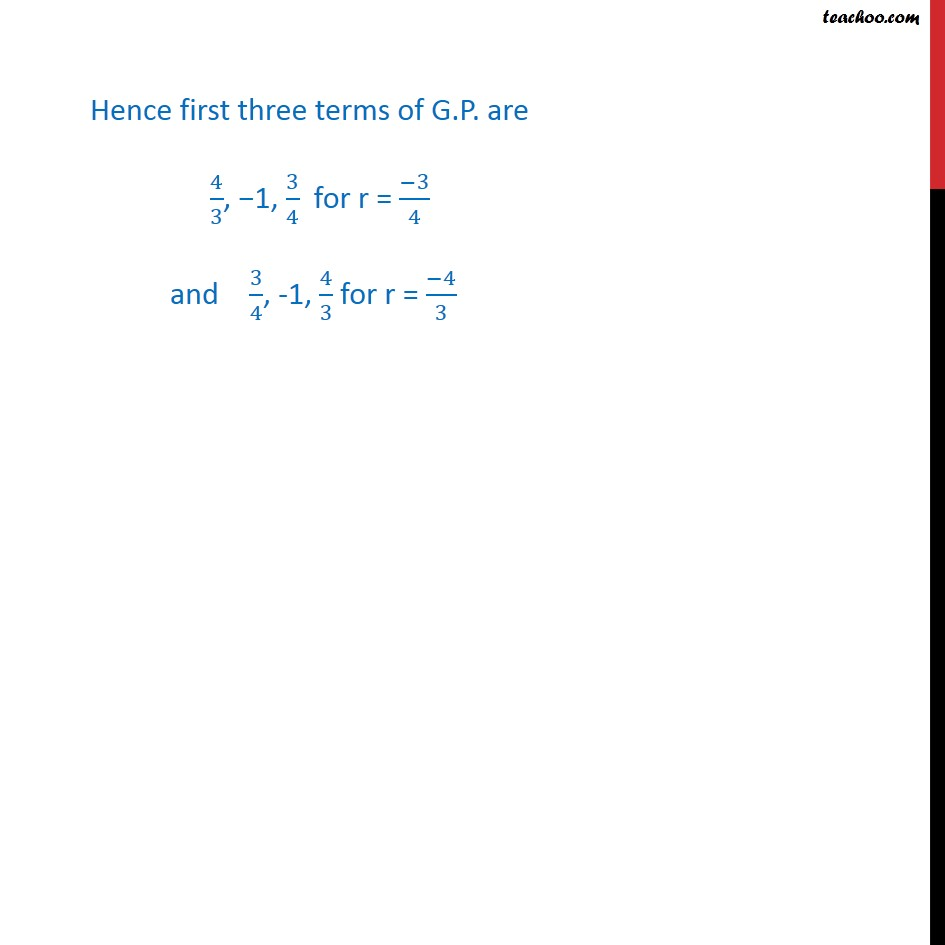Learn in your speed, with individual attention - Teachoo Maths 1-on-1 Class

### Transcript

Example 9 The sum of first three terms of a G.P. is 13/12 and their product is – 1. Find the common ratio and the terms. Let the three terms in G.P. be 𝑎/𝑟, a, ar here 1st term of G.P. = 𝑎/𝑟 2nd term of G.P. = a 3rd term of G.P. = ar It is given that Sum of first three terms of G.P. = 13/12 i.e. a/r + a + ar = 13/12 (− 1 −r −r2)/r = 13/12 12( -1 – r – r2 )= 13r -12 – 12r – 12r2 = 13r -12 - 12r - 12r2 – 13r = 0 -12 - 25r - 12r2 = 0 -(12 + 25r + 12r2)= 0 12r2 + 25r + 12= 0 This equation is of the form ax2 + bx + c = 0 where a = 12 b = 25 c = 12 & x = r